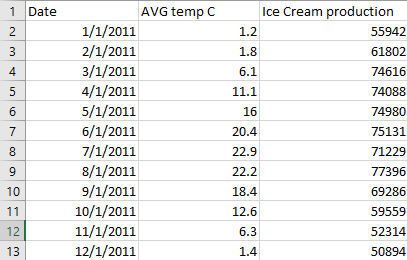# Create a correlation Matrix using Python

• Last Updated : 27 Jan, 2022

A correlation matrix is a table containing correlation coefficients between variables. Each cell in the table represents the correlation between two variables. The value lies between -1 and 1. A correlation matrix is used to summarize data, as a diagnostic for advanced analyses and as an input into a more advanced analysis. The two key components of the correlation are:

• Magnitude: larger the magnitude, stronger the correlation.
• Sign: if positive, there is a regular correlation. If negative, there is an inverse correlation.

A correlation matrix has been created using the following two libraries:

1. Numpy Library
2. Pandas Library

Method 1: Creating a correlation matrix using Numpy library

Numpy library make use of corrcoef() function that returns a matrix of 2×2. The matrix consists of correlations of x with x (0,0), x with y (0,1), y with x (1,0) and y with y (1,1). We are only concerned with the correlation of x with y i.e. cell (0,1) or (1,0). See below for an example.

Example 1: Suppose an ice cream shop keeps track of total sales of ice creams versus the temperature on that day.

## Python3

 `import` `numpy as np`  `# x represents the total sale in``# dollars``x ``=` `[``215``, ``325``, ``185``, ``332``, ``406``, ``522``, ``412``,``     ``614``, ``544``, ``421``, ``445``, ``408``],` `# y represents the temperature on``# each day of sale``y ``=` `[``14.2``, ``16.4``, ``11.9``, ``15.2``, ``18.5``, ``22.1``,``     ``19.4``, ``25.1``, ``23.4``, ``18.1``, ``22.6``, ``17.2``]` `# create correlation matrix``matrix ``=` `np.corrcoef(x, y)` `# print matrix``print``(matrix)`

Output

```[[1.         0.95750662]
[0.95750662 1.        ]]```

From the above matrix, if we see cell (0,1) and (1,0) both have the same value equal to 0.95750662 which lead us to conclude that whenever the temperature is high we have more sales.

Example 2: Suppose we are given glucose level in boy respective to age. Find correlation between age(x) and glucose level in body(y).

## Python3

 `import` `numpy as np`  `# x represents the age``x ``=` `[``43``, ``21``, ``25``, ``42``, ``57``, ``59``]` `# y represents the glucose level``# corresponding to that age``y ``=` `[``99``, ``65``, ``79``, ``75``, ``87``, ``81``]` `# correlation matrix``matrix ``=` `np.corrcoef(x, y)``print``(matrix)`

Output

```[[1.        0.5298089]
[0.5298089 1.       ]]```

From the above correlation matrix, 0.5298089 or 52.98% that means the variable has a moderate positive correlation.

Method 2: Creating correlation matrix using Pandas library

In order to create a correlation matrix for a given dataset, we use corr() method on dataframes.

Example 1:

## Python3

 `import` `pandas as pd`  `# collect data``data ``=` `{``    ``'x'``: [``45``, ``37``, ``42``, ``35``, ``39``],``    ``'y'``: [``38``, ``31``, ``26``, ``28``, ``33``],``    ``'z'``: [``10``, ``15``, ``17``, ``21``, ``12``]``}` `# form dataframe``dataframe ``=` `pd.DataFrame(data, columns``=``[``'x'``, ``'y'``, ``'z'``])``print``(``"Dataframe is : "``)``print``(dataframe)` `# form correlation matrix``matrix ``=` `dataframe.corr()``print``(``"Correlation matrix is : "``)``print``(matrix)`

Output:

```Dataframe is :
x   y   z
0  45  38  10
1  37  31  15
2  42  26  17
3  35  28  21
4  39  33  12
Correlation matrix is :
x         y         z
x  1.000000  0.518457 -0.701886
y  0.518457  1.000000 -0.860941
z -0.701886 -0.860941  1.000000```

Example 2:

CSV File used:## Python3

 `import` `pandas as pd`  `# create dataframe from file``dataframe ``=` `pd.read_csv(``"C:\\GFG\\sample.csv"``)` `# show dataframe``print``(dataframe)` `# use corr() method on dataframe to``# make correlation matrix``matrix ``=` `dataframe.corr()` `# print correlation matrix``print``(``"Correlation Matrix is : "``)``print``(matrix)`

Output:

```Correlation Matrix is :
AVG temp C  Ice Cream production
AVG temp C              1.000000              0.718032
Ice Cream production    0.718032              1.000000```

My Personal Notes arrow_drop_up